Your research can change the world
More on impact ›

# Frontiers in Applied Mathematicsand Statistics## ORIGINAL RESEARCH article

Front. Appl. Math. Stat., 09 April 2019 | https://doi.org/10.3389/fams.2019.00016

# Chimera and Anticoordination States in Learning DynamicsHaydée Lugo1*,Juan Carlos González-Avella2 and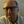Maxi San Miguel3
• 1ICAE, Department of Economic Analysis, Complutense University of Madrid, Madrid, Spain
• 2APSL S.L, Edifici Europa - Planta baja Galileo Galilei, Palma de Mallorca, Spain
• 3IFISC (CSIC-UIB), Campus Universitat Illes Balears, Palma de Mallorca, Spain

In many real-life situations, individuals are dared to simultaneously achieve social objectives of acceptance or approval and strategic objectives of coordination. Since these two objectives may take place in different environments, a two-layer network is the simple and natural framework for the study of such kind of dynamical situations. In this paper we present a model in which the state of the agents corresponds to one of two possible strategies. They change their states by interaction with their neighbors in the network. Inside each layer the agents interact by a social pressure mechanism, while between the layers the agents interact via a coordination game. From an evolutionary approach, we focus on the asymptotic solutions for all-to-all interactions across and inside the layers and for any initial distribution of strategies. We find new asymptotic configurations which do not exist in a single isolated social network analysis. We report the emergence and existence of chimera states in which two different collective states coexist in the network. Namely, one layer reaches a state of full coordination while the other remains in a dynamical state of coexistence of strategies. In addition, the system may also reach a state of global anticoordination where a full coordination is reached inside each layer but with opposite strategies in each of the two network layers. We trace back the emergence of chimera states and global anticoordination states to the agents inertia against social pressure, referred here to as the level of skepticism, along with the degree of risk taken into account in a general coordination game.

## 1. Introduction

The chimeric states refer to the emergence of a hybrid state in a coupled dynamic system in which one domain of the system exhibits a coherent behavior in combination with another domain that displays an incoherent behavior. The coexistence of coherent and incoherent states has received much attention as an intriguing manifestation of collective behavior. This interesting behavior was first observed by Kuramoto et al.  and then named it as chimera state . Although the literature about chimera states started with the study of interacting populations of oscillators in dynamical systems , it has been dizzily expanded to many fields in physics, chemistry, biology, etc (see ). Also in social systems, situations of two interacting populations in which one exhibits a coherent or synchronized behavior while the other is incoherent or desynchronized are commonly observed [21, 22]. This phenomena has also been addressed from the conceptual framework of chimera states. Models based on individual interactions have been introduced for opinion formation and cultural dissemination  and  in order to analyze the emergence of localized coherent or chimeras states behavior in social contexts. The systems considered consist in two populations of social agents mutually coupled through global interaction fields that account for the state of the majority of the agents in each population. The internal interactions in each group have a condition that allow for non-interacting states. Two examples of such dynamics have been analyzed: (i) Axelrod's model for social influence , and (ii) a discrete version of a bounded confidence model for opinion formation . In both systems, there are localized coherent states for some parameter values, in which a group reaches a homogenous or consensus state, while the other group remains in a disordered or polarized state. In this paper we contribute further to the study of chimera states in social systems, searching for them in the context of models of social coordination and learning dynamics .

The goal of reaching coordination has become one of the most important challenges in modern societies: In spite of the fact that individuals are now more connected and handle more information due to technological progress, it seems that coordination to reach consensus is becoming an increasingly difficult goal. It is common to observe how some societies become polarized either by economics concerns, ideological or political opinions, or, the collective behavior leads the population to states in which one part reaches a consensus while the other part behaves in an unstable manner, or it displays a dynamical coexistence of states. It can be argued that such diversity of outcomes is the result of a constant search for achieving simultaneously two different kinds of aims, namely, social and economic aims. On one side, individuals, influenced by others, seek for social acceptance and recognition and on the other side they attempt to get higher gains. Whenever the social and economic concerns take place in two different social networks or environments, the population unavoidably splits into two disjoint target groups. For instance, we can consider a country divided into two regions. Between the regions the habitants search for fulfilling economic aims and inside each region they search for social aims of approval and acceptance.

Here we present a simple model to illustrate such situation in which individuals of a population divided into two groups are dared to coordinate in order to accomplish their social and economic goals. Our framework for studying such kind of population is a two-layer network. Each layer corresponds to a group of the population and the interactions within the group aim to satisfy social concerns, while interactions between agents of different layers aim to satisfy economic concerns. In our system, the economic goal turns out to be reached when agents play the same strategy. Since there will be as many consensus as numbers of possible strategies a coordination problem arises. This situation is an important issue in economics, being analyzed in many theoretical and experimental studies. From the game-theoretical approach this situation has being modeled as a non-cooperative game called coordination game, . In our framework, the individuals play a pairwise two-person two-strategy coordination game.

In a social context, there is a wide number of theoretical models and empirical evidences that explain and show how the social influence can lead individuals to modify their behaviors, attitudes or beliefs, and as results, collective behaviors of consensus, polarization, or diversity may arise, , , . Here, we consider an alternative model of social influence based on the popularity of the strategies instead of the payoffs. Agents search for social goals of acceptance or approval of the strategy they use in their interlayer interactions.

There are a number of studies on population games with binary choices and externalities in discrete time (e.g., ). Within this literature, an interesting recent contribution by Dal Forno and Merlone  considers the dynamical effect in discrete time of a reference group in a system of two-group population. They consider the reference group as a “model” in which the behavior of such group affects the other population's dynamics. Our study follows the two-group population model described in Lugo and San Miguel . Both models study the dynamics of a system of two-group population with binary choices in discrete time and obtain results that can not be obtained in a single isolated system. However, our model differs from Dal Forno and Merlone  in several aspects, in particular in two main points. A first one is where the binary choice game takes place. In our model individuals in one population play the two-strategy coordination game with the individuals in the other population, instead of playing a game inside each population as in the model of Dal Forno and Merlone . A second main difference is where the social influence takes place. In our model, it takes place inside each population. In terms of the approach of Dal Forno and Merlone , we may say that each group serves as its own reference group.

Despite the simplicity of our model, the accomplishment of social goals can be difficult to achieve because there is skepticism in people to be influenced by the popularity of the opposite strategy of their partners. The previous work of Lugo and San Miguel  that considers such population searching for social and strategic objectives shows that for an initial uniform distribution of two possible strategies the skepticism to follow the opposite strategy and the local connectivity are the driving forces to accomplish full coordination for this two-layer network. Here, we consider the role of different initial conditions leading to different asymptotic states of the dynamics and we determine the basin of attraction of these states. As one of our main results, we find two asymptotic states with non-trivial collective behavior which can not be found in a single isolated network analysis. A first outcome is a social analog of a chimera state with coexistence of coherent and incoherent states. In our model, one layer can reach a homogeneous state of full coordination while the other remains in a dynamical state of coexistence of strategies. The second non-trivial asymptotic state is the anticoordination or polarization state in which the system reaches coordination with a different strategy in each layer.

The paper is organized as follows. Section 2 introduces the general frame of our model. Based on a two-layer network, we describe the kind of interactions inside and between layers and the dynamical rule for individuals to update their strategies. Section 3 describes the possible asymptotic solutions of the collective dynamics reached by the system, as well as the basins of attraction to reach these solutions. In particular we describe the non-trivial chimera and anticoordination states and their basins of attraction. Section 4 shows bifurcation diagrams, in section 4.1 for the case of a symmetric coordination game and in section 4.2 for the case of an asymmetric coordination game. Finally, section 5 summarizes our conclusions. In the Appendix, we present a mean-field theory for the time evolution of the system in the infinite size limit.

## 2. Methods

We consider an individual based model consisting in a population in which individuals interact in two different groups, A and B of sizes NA and NB respectively. We take NA = NB = N. Using the frame of a two-layer network (see Figure 1), inside each layer the individuals interact with social objectives and between layers they interact with strategic or economic objectives, as described in the following.

FIGURE 1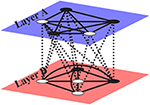Figure 1. Sketch of a two-layer network. The nodes are connected to each other in a pairwise manner both inside the layers (intralayer links) and across the layers A and B (interlayer links). Dotted lines describe the interlayer interactions and the solid lines describe the intralayer interactions. Black nodes stand for the agents playing strategy L, while white nodes stand for the agents playing strategy R in a coordination game.

### 2.1. Interactions Between the Layers: Coordination Games

In Game Theory, the coordination game is a prototype model of a non-cooperative game in which players share the goal of coordinating on any of the feasible actions, . It has multiple pure strategy Nash equilibria, and hence, a problem of equilibrium selection arises. In our model, players between the layers play a two-person, two-strategy coordination game.

The strategic interaction proceeds as follows. In each time step, each agent in a layer plays with each agent in the other layer a pairwise coordination game. Table 1 shows the payoff normal form representation of a coordination game: For example if one agent plays L and the other plays R, the payoff for the former is 0, and −b for the latter. We focus our analysis on two parametric settings, a pure or symmetric coordination game (SCG) in which s = 0 and b = 0 and a general or asymmetric coordination game (ACG) in which s = 1 and b > 0. The profiles (L, L) and (R, R) are the two pure strategy Nash equilibria in both settings. A problem of equilibrium selection is present in both settings. In the symmetric coordination game both equilibria are equivalent, in the sense that the payoff of each player for coordinating either on L or R is equal to 1. However, in the general coordination game, the payoff is equal to 2 for coordinating on (R, R) and 1 for coordinating on (L, L). Then, the higher payoff is achieved when agents coordinate on R. The profile (R, R) is the socially efficient solution and it is known in game theory, as the Pareto (payoff) dominant equilibrium. However, when b > 1, the profile (L, L) becomes the risk dominant equilibrium, in the sense of . When players coordinate on (L, L), the cost of a unilateral deviation is 1+b and when they coordinate on (R, R) the cost of a unilateral deviation is 2. The cost of deviation from L to R is greater than the cost of deviation from R to L, if 1+b > 2, or equivalently, if b > 1. In this case, by the criterion of risk dominance, when b > 1, the agents select the profile (L, L) since the socially efficient solution (R, R) turns risky. The parameter b becomes a measure of the risk for playing the strategy R. For a complete review, see .

TABLE 1

### 2.2. Interactions Inside the Layers: the Effect of Social Pressure

Inside each layer, searching for social acceptance and approval, each agent observes the strategies being played by her partners. An agent may not feel at ease with her strategy when such strategy is not as popular as she wants in her social environment. The level of skepticism in the population is calibrated by a threshold T that determines the effect of the social pressure exerted on an individual. The criterion used by each player i is to measure how well she is doing by comparing the share of agents who are playing the opposite strategy to hers, denoted by di, with the threshold T ∈ [0, 1]. We may distinguish two types of populations. Herding population, for T < 0.5, in which individuals are influenced by low levels of popularity of the opposite strategy, and, skeptical population, for T > 0.5, where the social pressure has a weak effect on individuals: a feeling of disapproval only arises for high levels of popularity of the opposite strategy. Therefore, when di>T, the social pressure is effective because player i generates a feeling of non-acceptance about the strategy she is currently playing and she is willing to revise it.

### 2.3. Inter-layer and Intra-layer Objectives: The Degree of Satisfaction

In the interactions across and inside the layers agents intend to satisfy two different objectives: social objectives of acceptance and approval, and strategic objectives of coordination. These objectives give rise into two different sources of satisfaction: strategic satisfaction in terms of the monetary payoff obtained in the coordination game and social satisfaction in terms of the popularity of the current strategies. Therefore, there are four degrees of satisfaction described in Table 2, where πi is the aggregate payoff that agent i in a layer gets playing with all the other agents in the other layer.

TABLE 2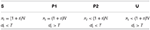Table 2. Degrees of satisfaction according to the fulfilment of social and strategical objectives.

The value of s is derived from the parametric setting of the coordination game. When s = 0 the equality πi = N shows the total payoff when player i coordinates with all the members of the other layer in the symmetric game. Then we say that agent i is strategically satisfied. In the case of a general coordination game, s = 1, an agent is strategically satisfied when the coordination is on the socially efficient solution, i.e., the Pareto dominant strategy. Then, the total payoff is πi = 2N. Besides, when di < T the share of agents inside the layer of player i who play the same strategy as she does is high enough so the player i feels socially satisfied with her current strategy. This means that her social objectives of acceptance and approval are fulfilled. Then, the level of satisfaction of agent i can be: S (satisfied) when she is both socially (di < T) and strategically (πi = (1+s)N) satisfied, P1 or P2 (partially satisfied) when she is either socially (di < T) or strategically (πi = (1+s)N) satisfied and is U (unsatisfied) when she is both socially (di > T) and strategically (πi < (1+s)N) unsatisfied.

### 2.4. Learning Dynamics

The learning dynamics in the system is described by the update rule used to change strategy: at each elementary time step each player plays the coordination game with all the members in the other layer. Once the game is over and an aggregate payoff is assigned to each player, each agent observes and measures the popularity of her strategy in her own group. As a result, a level of satisfaction arises. Then, she might change her strategy impelled by her level of satisfaction. The process is repeated setting aggregate payoffs to zero. The synchronous update rule in which each player can change her current strategy according to her level of satisfaction is described as follows,

1. If her level of satisfaction is S, she remains with the same strategy.

2. If her level of satisfaction is P1 or P2, she imitates the strategy of her best performing agent inside the layer in case that such agent has received a larger payoff than the player herself, otherwise she remains with the same strategy.

3. If her level of satisfaction is U, she changes her current strategy.

Although the update rule takes place inside the layers, individuals change their strategies by both social and strategic considerations. The imitation of the best performing individuals in her social environment aims to capture the individual behavior observed in many complex real life situations. This learning dynamics was first implemented in Lugo and San Miguel  to study the effect of local interactions on a two-layer network with an initial uniformly distribution of strategies and also, in González-Avella et al.  to study the emergence of polarization in a skeptical population for any initial distribution of strategies.

## 3. Results

### 3.1. Asymptotic Solutions

Analytical equations for our model and their asymptotic solutions are discussed in the Appendix. For the general case of the asymmetric coordination game these solutions, described below, are the following:

Solution I: Coordination in strategy L: All agents in both layer play strategy L. It is linearly stable and exists for any T ∈ [0, 1].

Solution II: Coordination in strategy R: All agents in both layer play strategy R. It is linearly stable and exists for any T∈[0, 1].

Solutions III: Coexistence of strategies. These solution exist for any T≠1 and it occurs in two ways:

(1) unstable fixed points and,

(2) family of marginally stable periodic solutions.

Solutions III-a and III-b: Chimera solutions. This is an interesting case of coexistence of two distinct solutions, namely, solutions I and III. One of the layers goes to the absorbing state of coordination in strategy L, namely layer A for Solution III-a and layer B for solution III-b, while the other layer goes to a dynamical state of coexistence of strategies, solution III type (2). We also find the case of coexistence of two solutions in which one layer coordinates in L and the other layer remains disordered with both strategies coexisting in the same proportion [Solution III type (1)]. Chimera solutions only appear when agents are playing the asymmetric coordination game. They exist for almost any T < 0.5 and almost any b>0.

Although the strategies L and R are not equivalent in the asymmetric coordination game because the first is the socially undesired and the second the socially desired outcome, solutions III-a and III-b are equivalent in the sense that the layer reaching the absorbing state L is determined by the initial conditions of strategies in the two layers. We refer to these solutions as chimera states because of the coexistence of an ordered layer and a disordered layer. The disordered layer can be in a dynamical state [solution III (2)] or in a configuration in which the number of agents playing each strategy is equal and constant in time [solution III (1)].

Solutions IV Anticoordination states, layer A coordinates in strategy L while layer B in strategy R.

Solutions V Anticoordination states, layer A coordinates in strategy R while layer B in strategy L.

Solutions IV and V exist and they are linearly unstable and exist for almost all T ∈ [0.5, 1].

We summarize in Figure 2 the domain of existence of the different asymptotic solutions for the general case of the asymmetric coordination game as a function of the threshold T and the risk b parameters. For this general case the parameters of the pay-off matrix take the values s = 1 and b>0 for any T∈[0, 1]. Asymptotic solutions of the dynamics depend on initial conditions (see below Figure 5), and in this sense we refer to Q1 as those chimera states, solutions III-a and III-b, that are reached from initial conditions such that ${{x}_{aa}}^{0}+{{x}_{bb}}^{0}<1$, where ${{x}_{aa}}^{0}$ and ${{x}_{bb}}^{0}$ are the initial conditions for xaa and xbb respectively. Likewise we refer to Q2 as those chimera states, solutions III-a and III-b, that are reached from initial conditions such that ${{x}_{aa}}^{0}+{{x}_{bb}}^{0}>1$. It turns out that solutions Q1 exist for b>0, zones A, C, and D in Figure 2, while Q2 only exist for b>1, zones D and C. The range of values of b and T in which the system reaches solutions Q1, Q2, I ,II and III corresponds to zones C and D in the Figure. The difference between zones C and D refers to the areas of the basins of attraction of each solution, as explained in section 3.2. For the case of symmetric coordination game, the parameters take the values s = 0 and b = 0. The asymptotic solutions in this case are the same as those for the asymmetric coordination game except for Solutions III-a and III-b, the Chimera states. Chimera states could not be found for any level of skepticism in the case of the symmetric coordination game.

FIGURE 2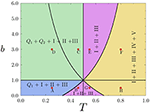Figure 2. Domain of existence of the different asymptotic solutions in the Asymmetric Coordination Game (ACG) as a function of the threshold T and the risk parameter b as obtained from the analytic solution of the equations discussed in the Appendix. The two curves ($T=\frac{1+b}{3+b}$ and $1-T=\frac{1+b}{3+b}$) and the two lines (b = 1, T = 0.5) divide the figure in eight zones from (A) to (H). In each of these zones the asymptotic solutions that exist are indicated. The red dots correspond to values of T and b used in Figure 5.

Given that the agent population is divided in two layers, A and B, we define xaa as the fraction of individuals playing strategy R in layer A, and xbb as the fraction of individuals playing strategy R in layer B. To describe the different asymptotic solutions we also introduce the order parameter nAB giving the density of inter-layer active links, i.e., the proportion of links connecting agents in different layers with opposite strategies. The order parameter nAB can be written in terms of xaa and xbb by,

$nAB=xaa(1-xbb)+xbb(1-xaa)$

The different solutions described in terms of the asymptotic values of nAB, xaa, and xbb are shown in Table 3. These solutions follow from the mean-field analysis described in the Appendix. We next describe these solutions as obtained from numerical simulations. In these simulations, we have fixed the system size NA+NB = 2000 where NA = NB = 1000.

TABLE 3

Figure 3 shows the time evolution of xaa and xbb, as obtained from the simulation of our individual based model, for different initial conditions that lead to the asymptotic solutions I, II, III, III-a, III-b, IV, and V. Figures 3A–G correspond to the symmetric coordination game (SCG), while chimeras states appearing in the asymmetric coordination game (ACG) are shown in Figures 3H–J. In Figures 3A,B the order parameter nAB = 0, indicates that the system goes to an absorbing state in which the agents in both layers play the same strategy. Figure 3A shows that after a short transient time the fractions xaa = xbb = 0 and the density of inter-layer active links is nAB = 0 corresponding to solution I, while for Figure 3B after a short transient time the fractions xaa = xbb = 1 and nAB = 0 corresponding to solution II.

FIGURE 3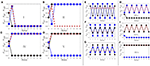Figure 3. Time evolution of xaa (circles), xbb (squares) and the density of active inter-layer links nAB (dashed lines). (A–D) Show the time evolution to coordination (solutions I and II) and anticoordination states (solutions IV and V) for symmetric game (s = 0, b = 0) with T = 0.75. (E–G) Show the phase and anti-phase oscillations [solutions III (2)] of the fraction of strategies xaa and xbb for symmetric game (s = 0, b = 0) with T = 0.25. (H–J) Show the temporal evolution of xaa and xbb and the active inter-layer links nAB, for the asymmetric coordination game (s = 1, b = 0.5) with T = 0.25. (H,I) Show the chimera states, solution III-a and III-b respectively and (J) shows the case when the initial and the final states are the same when xaa = 0 and xbb = 0.5.

In Figure 3C (solution IV) and Figure 3D (solution V), the value nAB = 1 indicates that in both cases the system goes to an anticoordination absorbing state, i.e., agents in each layer are playing opposite strategies. This state of anticoordination can emerge only in skeptical populations where T>0.5. It is interesting to notice that the layer with an initial higher proportion of R is the layer that ends playing L. A complete analysis of the anticoordination solutions for a skeptical two-group population can be found in González-Avella et al. .

It is important to note that there exist absorbing states other than solutions I, II, IV, and V. They correspond to an unstable fixed point xaa = xbb = r of the dynamics for 0 < r < 1. These solution correspond to the classification (1) of Solution III, namely, r = 1/2 in the SCG for all T∈(0, 1) and in the ACG for T < 1/2, or the fixed point $r=\frac{1+b}{3+b}$ in ACG when T>1/2.

Figures 3E–G display the temporal evolution of the system for marginally stable periodic solutions [solutions III (2)] in the case of the symmetric coordination game. The asymptotic dynamical configurations show phase (Figures 3F,G) and anti-phase (Figure 3E) oscillations of strategies between the two layers. Note that 0 < nAB < 1 remains constant during these oscillations.

The Chimera solutions are illustrated in Figures 3H–J for the ACG with parameter values T = 0.25, b = 0.5. Figure 3H corresponds to a solution III-a, in which all agents play strategy L in layer A, i.e., xaa = 0 while a configuration of dynamical coexistence of strategies takes place in layer B, i.e., 0 < xbb < 1. Note that the fraction of agents that choose to play strategy L or R in layer B changes over time, so that we also observe an oscillation of nAB. Figure 3I corresponds to a solution III-b. In this case the behavior is similar to the one in Figure 3H, but now the L coordinating absorbing state occurs in layer B, while the dynamical coexistence of strategies appears in layer A. We also notice that possibly similar solutions with xaa≠0 constant, and xbb oscillating are not found in our model due to the non-equivalence of the L and R strategies in the ACG. In Figure 3J we illustrate the particular case of a chimera state in which one layer coordinates in L, in this case layer B, while the other layer remains in a solution III (1) in which agents in that layer start and continue playing for all times both strategies with equal proportions.

The particular collective behaviors described by solutions III-a and III-b, are the social analog of a chimera state arising in two interacting populations of oscillators observed in dynamical systems. In general a chimera state describes a situation where two populations that interact with each other, one exhibits a coherent or synchronized behavior while the other is incoherent or desynchronized. Likewise we have two populations of interacting agents such that one reaches an absorbing state, while the other remains in a dynamically disordered state. The chimera states only arise in our system when the population is herding (T < 0.5) and play an asymmetric coordination game. This means that beside the initial distribution of strategies, a herding behavior is the underlying mechanism that allows to reach chimera states when the two Nash equilibria of the coordination game are not equivalent in terms of payoff.

### 3.2. Basins of Attraction: The Global Picture

Depending on the initial conditions for xaa and xbb, the system reaches different asymptotic solutions characterized by their value of the order parameter nAB. Extensive numerical simulations of our individual based model are summarized in Figures 4, 5 that show the basins of attraction of the different asymptotic solutions in terms of the initial densities of xaa and xbb, and for different values of the threshold parameter T for the SCG and for different values of T and b for the ACG, respectively. The color code defines the solutions in terms of the fraction of inter-layer active links. Both figures show how the basins of attraction in terms of the initial conditions are determined by the value of the threshold parameter T in the case of the SCG and the threshold T along with the parameter b in the case of the ACG.

FIGURE 4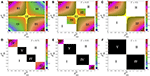Figure 4. Plot in color scale of the fraction of active links between layer A and B in the asymptotic solution of the dynamics as a function of the initial density of xaa and xbb for the Symmetric Coordination Game (s = 0, b = 0). The color scale defines the values of the fraction of actives links, nAB = 1 black color and nAB = 0 white color. Asymptotic solutions are as indicated. (A–F) Correspond to different values of T: (A) T = 0, (B) T = 0.25, (C) T = 0.5, (D) T = 0.75, (E) T = 0.85, and (F) T = 1. System size, NA+NB = 1000+1000 = 2000.

FIGURE 5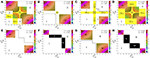Figure 5. Plot in color scale of the fraction of active links between layer A and B in the asymptotic solution of the dynamics as a function of the initial density of xaa and xbb for the Asymmetric Coordination Game. The color scale defines the values of the fraction of actives links, nAB = 1 black color and nAB = 0 white color. Asymptotic solutions are as indicated. (A–H) Correspond to the values of the threshold T and parameter b indicated by red dots in Figure 2. (A) T = 0.25, b = 0.50, (B) T = 0.46, b = 0.50, (C) T = 0.25, b = 0.30, (D) T = 0.25, b = 3.00, (E) T = 0.55, b = 3.00, (F) T = 0.80, b = 3.00, (G) T = 0.55, b = 0.50, (H) T = 0.80, b = 0.50. System size, NA+NB = 1000+1000 = 2000.

## 4. Bifurcation Diagrams

In this section we consider possible transitions among the different solutions discussed before. These transitions are described by means of bifurcations diagrams obtained in terms of the control parameters T and b.

### 4.1. Symmetric Coordination Game

We have shown in the previous section that the solution obtained for the SCG, and for a fixed initial condition, depends on the value of the threshold parameter T, so that by varying T we find transitions among those solutions. Examples of these transitions are shown in Figures 6A–C. These are bifurcation diagrams that give the average of the fractions of inter-layer active links nAB or 1−nAB as a function of the threshold T. These bifurcation diagrams describe transitions that occur, for threshold values of T, between solutions III to I, III to V, and I to V, respectively for different fixed values of the initial conditions. Each panel shows two examples. We also find subsequent transitions among three solutions. For instance, the bifurcation diagram Figure 7A for the average of nAB, illustrates a first transition between solution III and solution I, followed by a second transition between I and V as T increases. These results show the effect of the skepticism on the collective behavior in a two-layer network. Tuning the level of skepticism from the limit value T = 0, where the population is extremely herding to the limit value T = 1 where the population is extremely skeptical. Figure 4 indicates that the system goes from a state of complete coexistence of strategies, Solution III for almost all initial conditions, to states of anticoordination, Solutions IV and V, and states of global coordination, Solutions I and II.

FIGURE 6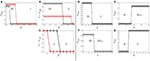Figure 6. Bifurcation diagrams for the average of nAB as function of T for fixed initial conditions for the SCG, (A–C), and for the ACG, (D–G). (A): xaa = 0.40 and xbb = 0.10 (circles), xaa = 0, 40 and xbb = 0.30 (squares). (B): xaa = 0.40 and xbb = 0.60 (circles), xaa = 0, 10 and xbb = 0.90 (squares). (C): xaa = 0.25 and xbb = 0.51 (circles), xaa = 0, 37 and xbb = 0.52 (squares). (D): xaa = 0.30 and xbb = 0.45 with T = 0.75. (E): xaa = 0.10 and xbb = 0.48 with T = 0.15. (F): xaa = 0.25 and xbb = 0.1 with T = 0.7. (G): xaa = 0.45 and xbb = 0.65 with T = 0.75.

FIGURE 7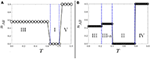Figure 7. (A): Bifurcation diagram among solutions III, I, and V of the average of nAB as function of T in the SCG for a fixed initial condition xaa = 0.20 and xbb = 0.60. (B): Bifurcation diagram among solutions III, III-a, II, and IV of the average of nAB as function of T for a fixed initial condition xaa = 0.78 and xbb = 0.60 where the risk parameter b = 3 in the ACG.

### 4.2. Asymmetric Coordination Game

As discussed before, Figure 2 shows for the ACG case a phase diagram, obtained from a mean field theoretical approach, indicating domains of existence of different asymptotic solutions in the b- T parameter space. In comparison with the SCG case, the additional parameter b allows for new transitions that occur for a threshold value of b and fixed T, including transition to chimera states. Examples of these transitions are shown in Figures 6D–G. Figure 6D shows a transition between a state of anticoordination IV and a state of full coordination I and Figure 6G shows a transition between a state of full coordination II and a state of anticoordination V, while Figures 6E,F show transitions between a state of coordination II or dynamical coexistence III and a chimera state III-a. On the other hand, Figure 7B shows an example of subsequent transitions as T increases for fixed b = 3, with a first transition between a state of dynamical coexistence III and a chimera state III-a, followed by a transition between III-a and a state of full coordination II and a final transition between II and a state of anticoordination IV.

A different form of bifurcation diagrams can be obtained by considering the area of the basin of attraction of a given solution in the parameter space of the initial conditions ${{x}_{aa}}^{0}$ and ${{x}_{bb}}^{0}$. Results for this area are indicated in Table 4 for the chimera states Q1 and Q2 and the different zones of the T-b parameter space of Figure 2. We recall that Q1 are those solutions III-a and III-b reached from initial conditions such that ${{x}_{aa}}^{0}+{{x}_{bb}}^{0}<1$, while Q2 are those obtained when ${{x}_{aa}}^{0}+{{x}_{bb}}^{0}>1$. The areas of the basins of attractions AQ1, AQ2 for Q1 and Q2 respectively are plotted vs. T and b in Figure 8. Using AQ1, AQ2 as order parameters, these figures show bifurcation diagrams for the transition from existence AQ≠0 to non-existence AQ = 0 of a chimera state.

TABLE 4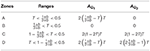Table 4. Areas of the basin of attraction of chimera states in the parameter space of initial conditions of xaa and xbb according to the zones described in Figure 2.

FIGURE 8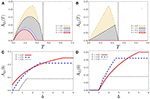Figure 8. Sizes of the basins of attraction of chimera states categorized by Q1 and Q2, denoted by AQ1 and AQ2, as function of T for different values of b (A,B) and as function of b for different values of T (C,D).

Figures 8A,B show a threshold value T = 0.5, so that chimera states exist for T < 0.5 in agreement with the phase diagram of Figure 2. They also show that as T increases the areas of the basin of attraction of chimera states first increase until a certain value of T and then they decrease to become zero for T = 0.5. In addition Figures 8C,D identify a threshold value of b for the existence of chimera states. For Q2 chimeras this is fixed at b = 1 independently of T, while for Q1 it depends on T, with Q1 chimera states existing for all values of b and T small enough. For both Q1 and Q2 we also identify a characteristic T-dependent value of b beyond which the area of the basin of attraction remains constant.

More generally and on a qualitative basis, it follows from Figures 4, 5 that, for any fixed b, the basins of attraction of solution III and chimeras states disappear as T increases, so that and in the limiting case of T = 1, only Solutions I, II, IV and V can be reached by the system. Another interesting limiting case is the one of the risk parameter b → ∞, where it is extremely risky to play strategy R. It can be expected that in this limit solution I becomes preponderant. Indeed, we show in Figure 9, as compared with Figure 5, that the basin of attraction of solution I increases, solution II disappears and solution III and chimeras remain for fixed values of T < 0.5. When both parameters b and T increase, solutions II, III, IV, and V disappear and the basin of attraction of solution I increases. In the limit case, T = 1 and b → ∞, solution I becomes the main solution in the system for almost every initial condition.

FIGURE 9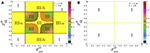Figure 9. Plot in color scale of the fraction of active links between layer A and B in the asymptotic solution of the dynamics as a function of the initial density of xaa and xbb for the Asymmetric Coordination Game. The color scale defines the values of the fraction of actives links, nAB = 1 black color and nAB = 0 white color. Asymptotic solutions are as indicated. (A): b = 100, T = 0.25, and (B): b = 100, T = 0.50.

## 5. Conclusions

We have considered a model of evolutionary game of a population divided into two groups where individuals are searching to fulfil their social and strategic objectives. The frame for this situation has been a multilayer network of two layers. Interactions within each layer aim to fulfill social objectives associated with learning dynamics, while interactions across layer consist in a coordination game, therefore involving strategic objectives. Our analysis, based on a mean-field theoretical approach and corroborated by numerical simulations of the model, reveals the existence of collective behaviors commonly observed on nature but impossible to find on a single isolated network analysis. In our multilayer framework we find states different of those of global coordination or dynamical states of coexistence of the strategies. Namely, in the multilayer coordination challenge, anticoordination and chimera states solution emerge. In the former the dynamics of the system polarizes the population, with each layer coordinating in a different strategy. This can also happen in the asymmetric coordination game where the two strategies correspond to different Nash equilibria: the socially efficient or Pareto dominant, and the risk dominant equilibrium. In the chimera states one layer coordinates in the risk dominant equilibrium, while the second remains disordered, that is with coexistence of the two strategies. This coexistence can be time independent or in the form of periodic solutions.

In connection with the standard notion of chimera states in two populations of dynamical oscillators having global or long range interactions [2, 8, 14, 40], we note that in our evolutionary game theory framework we also have the basic ingredients of two non-linear dynamical systems which are globally coupled. In chimera states of coupled oscillators, one population is in a coherent state and coexists with the other population in an incoherent state. In our social analog of the chimera state we have interpreted the coordination states in one layer as a coherent or ordered state, while we identify the incoherent state with the layer that exhibits coexistence of the two strategies. In most cases this coexistence is of dynamical nature, being the disordered layer in an active dynamical state of oscillation between the two possible strategies. Our model only incorporates two possible individual states of the agents, but we envisage that in social models including more individual states or strategies, such as those in reference [21, 22], the disordered state would show a richer dynamical behavior, since the individual elements can dynamically visit a large number of possible states. In this case the disordered or incoherent population would have a dynamical behavior similar to those found in populations of dynamical oscillators.

We observe chimera states only for the asymmetric coordination game where the coherent state reached is in the socially least desired coordination state. For herding individuals, the presence of a degree of risk in coordinating on the socially efficient outcome has an effect on the emergence of chimera states. However, for skeptical individuals, the anticoordination states are present in both symmetric and asymmetric coordination games. While the presence of two layers in the network is a consequence of the type of interaction that individuals have inside and across the layers, the actual factors that play a key role for the existence of chimera and anticoordination states are the level of skepticism and the existence of a risk parameter on the coordination game.

In the context of coordination in social systems, our contribution brings a more realistic insight about the consequences of a collective behavior that makes a distinction between social and strategic objectives. This collective behavior may lead herding societies to chimera states and skeptical societies to polarized states of anticoordination.

## Author Contributions

All authors conceived the study and designed the numerical simulations, HL and JG-A performed the numerical and analytical calculations, all authors analyzed the results, wrote, and reviewed the paper.

## Conflict of Interest Statement

The authors declare that the research was conducted in the absence of any commercial or financial relationships that could be construed as a potential conflict of interest.

## Acknowledgments

HL acknowledges financial support from Ministerio de Economía y Competitividad (Spain) under Project No. ECO2016-75992-P. MS acknowledges financial support from Agencia Estatal de Investigación (AEI, Spain) and Fondo Europeo de Desarrollo Regional under Project ESoTECoS Grant No. FIS2015-63628-C2-2-R (AEI/FEDER,UE) and the Spanish State Research Agency, through the María de Maeztu Program for Units of Excellence in R&D (MDM-2017-0711).

## Supplementary Material

The Supplementary Material for this article can be found online at: https://www.frontiersin.org/articles/10.3389/fams.2019.00016/full#supplementary-material

## References

1. Kuramoto Y, Battogtokh D. Coexistence of coherence and incoherence in nonlocally coupled phase oscillators. Nonlinear Phenom Complex Syst. (2002) 5:380–5.

2. Abrams DM, Strogatz SH. Chimera states for coupled oscillators. Phys Rev Lett. (2004) 93:174102. doi: 10.1103/physrevlett.93.174102

3. Martens EA, Panaggio MJ, Abrams DM. Basins of attraction for chimera states: fast track communication. New J Phys. (2016) 18:022002. doi: 10.1088/1367-2630/18/2/022002

4. Rakshit S, Bera BK, Perc M, Ghosh D. Basin stability for chimera states. Sci Rep. (2017) 7:2412. doi: 10.1038/s41598-017-02409-5

5. Suda Y, Okuda K. Persistent chimera states in nonlocally coupled phase oscillators. Phys. Rev. E (2015) 92:060901. doi: 10.1103/physreve.92.060901

6. Panaggio MJ, Abrams DM. Chimera states: coexistence of coherence and incoherence in networks of coupled oscillators. Nonlinearity(2015) 28:R67–87. doi: 10.1088/0951-7715/28/3/r67

7. Kundu S, Majhi S, Bera BK, Ghosh D, Lakshmanan, M. Chimera states in two-dimensional networks of locally coupled oscillators. Phys Rev E. (2018) 97:022201. doi: 10.1103/PhysRevE.97.022201

8. Omelchenko I, Maistrenko Y, Hovel P, Scholl E. Loss of coherence in dynamical networks: spatial chaos and chimera states. Phys Rev Lett. (2011) 106:234102. doi: 10.1103/physrevlett.106.234102

9. Hizanidis J, Kouvaris Nikos E, Zamora-López G, Díaz-Guilera A, Antonopoulos CG. Chimera-like states in modular neural networks. Sci Rep. (2016) 6:19845. doi: 10.1038/srep19845

10. Banerjee T, Dutta PS, Zakharova A, Schöll E. Chimera patterns induced by distance-dependent power-law coupling in ecological networks. Phys Rev E. (2016) 94:032206. doi: 10.1103/PhysRevE.94.032206

11. Röhm A, Böhm F, Lüdge K. Small chimera states without multistability in a globally delay-coupled network of four lasers. Phys Rev E. (2016) 94:042204. doi: 10.1103/PhysRevE.94.042204

12. Bastidas VM, Omelchenko I, Zakharova A, Schöll E, Brandes T. Quantum signatures of chimera states. Phys Rev E. (2015) 92:062924. doi: 10.1103/PhysRevE.92.062924

13. Viennot D, Aubourg L. Quantum chimera states. Phys Lett A. (2016) 380:678. doi: 10.1016/j.physleta.2015.11.022

14. Tinsley MR, Nkomo S, Showalter S. Chimera and phase-cluster states in populations of coupled chemical oscillators. Nat Phys. (2012) 8:662–5. doi: 10.1038/nphys2371

15. Nkomo S, Tinsley MR, Showalter K. Chimera states in populations of nonlocally coupled chemical oscillators Phys Rev Lett. (2013) 110:244102. doi: 10.1103/PhysRevLett.110.244102

16. Rattenborg NC, Amlaner CJ, Lima SL. Behavioral, neurophysiological and evolutionary perspectives on unihemispheric sleep. Neurosci Biobehav Rev. (2000) 24:817–42. doi: 10.1016/S0149-7634(00)00039-7

17. Laing CR, Chow CC. Stationary bumps in networks of spiking neurons. Neural Comput. (2001) 13:1473. doi: 10.1162/089976601750264974

18. Sakaguchi H. Instability of synchronized motion in nonlocally coupled neural oscillators. Phys Rev E. (2006) 73:031907. doi: 10.1103/PhysRevE.73.031907

19. Panaggio MJ, Abrams DM. Chimera states: coexistence of coherence and incoherence in networks of coupled oscillators. Nonlinearity (2015) 28:R67. doi: 10.1088/0951-7715/28/3/R67

20. Yao N, Zheng Z. Chimera states in spatiotemporal systems: theory and applications. Int J Mod Phys. (2016) bf B30:1630002. doi: 10.1142/S0217979216300024

21. González-Avella JC, Cosenza MG, San Miguel M. A model for cross-cultural reciprocal interactions through mass media PLOS ONE. (2012) 7:e51035. doi: 10.1371/journal.pone.0051035

22. González-Avella JC, Cosenza MG, San Miguel M. Localized coherence in two interacting populations of social agents. Physica A. (2014) 399:24–30. doi: 10.1016/j.physa.2013.12.035

23. Axelrod R. The dissemination of culture: a model with local convergence and global polarization. J Conflict Res. (1997) 41:203–26. doi: 10.1177/0022002797041002001

24. Deffuant D, Neau F, Amblard G. Weisbuch. Mixing beliefs among interacting agents. Adv Complex Syst. (2000) 3:87–98. doi: 10.1142/S0219525900000078

25. González-Avella JC, Eguíluz VM, Marsili M, Vega-Redondo F, San Miguel M. Threshold learning dynamics in social networks. PLoS ONE. (2011) 6:e20207. doi: 10.1371/journal.pone.0020207

26. Ellison G. Learning, local interaction, and coordination. Econometrica. (1993) 61:1047–71. doi: 10.2307/2951493

27. Vega-Redondo F. Economics and the Theory of Games. Cambridge: Cambridge University Press (2003).

28. Goeree JK Holt CA. Coordination games. In: The Encyclopedia of Cognitive Science, Vol 2. L. Nagel, ed., London: Nature Publishing Group; McMillan (2002). p. 204–8.

29. Weidenholzer S. Coordination games and local interactions: a survey of the game theoretic literature. Games. (2010) 1:551–85. doi: 10.3390/g1040551

30. Harsanyi J, Selten R. A General Theory of Equilibrium Selection in Games.Cambridge, MA: The MIT Press (1988).

31. Flache A, Mas M, Feliciani T, Chattoe-Brown E, Deffuant G, Huet S, et al. Models of social influence: towards the next frontiers. J Artif Soc Soc Simul. (2017) 20:2. doi: 10.18564/jasss.3521

32. Flache A, Macy MW. Local convergence and global diversity: from interpersonal to social influence. J. Conflict Resol. (2011) 55:970–95. doi: 10.1177/0022002711414371

33. Friedkin NE, Johnsen EC. Social Influence Network Theory. New York, NY: Cambridge University Press (2011).

34. Bischi GI, Merlone U. Global dynamics in binary choice models with social influence. J Math Sociol. (2009) 33:277–302. doi: 10.1080/00222500902979963

35. Bischi GI, Gardini L, Merlone U. Impulsivity in binary choices and the emergence of periodicity. Discrete Dyn Nat Soc. 2009:407913. doi: 10.1155/2009/407913

CrossRef Full Text

36. Dal Forno A, Merlone U. Heterogeneous society in binary choices with externalities. Dyn Games Appl. (2018). p. 1–25. doi: 10.1007/s13235-018-0270-x

37. Dal Forno A, Merlone U. Reference group influence on binary choices dynamics. Decisions Econ Finan. (2018). 41:427–45. doi: 10.1007/s10203-018-0216-1

38. Lugo H, San Miguel M. Learning and coordinating in a multilayer network. Sci. Rep. (2015) 5:7776. doi: 10.1038/srep07776

39. González-Avella JC, Lugo H, San Miguel M. Coordination in a skeptical two-group population. J Econ Interact Coord. (2018) 14:203–14. doi: 10.1007/s11403-018-0223-x

40. Laing CR. Chimeras in networks of planar oscillators. Phys Rev E. (2010) 81:066221. doi: 10.1103/PhysRevE.81.066221

Keywords: multilayer network, coordination games, chimera states, anticoordination states, skepticism

Citation: Lugo H, González-Avella JC and San Miguel M (2019) Chimera and Anticoordination States in Learning Dynamics. Front. Appl. Math. Stat. 5:16. doi: 10.3389/fams.2019.00016

Received: 12 December 2018; Accepted: 19 March 2018;
Published: 09 April 2019.

Edited by:

Eckehard Schöll, Technische Universität Berlin, Germany

Reviewed by:

Ugo Merlone, University of Turin, Italy
Sajad Jafari, Amirkabir University of Technology, Iran

Copyright © 2019 Lugo, González-Avella and San Miguel. This is an open-access article distributed under the terms of the Creative Commons Attribution License (CC BY). The use, distribution or reproduction in other forums is permitted, provided the original author(s) and the copyright owner(s) are credited and that the original publication in this journal is cited, in accordance with accepted academic practice. No use, distribution or reproduction is permitted which does not comply with these terms.

*Correspondence: Haydée Lugo, hlugo@ucm.es